Scale-up Suite Help
Full Factorial
What is Full Factorial?

Full Factorial enables you to vary factors in your model and calculate their effect on one or more responses. For example, if you wanted to check the effect of varying the temperature and reagent on the yield, you could vary both factors by 10% and check the effect of this on the yield.  Simulations will be run at each level of each factor; e.g. with two factors and three levels of each, there will be nine simulations.

Full Factorial can be accessed from the Dynochem ribbon: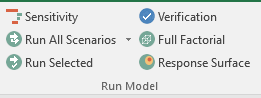New in Dynochem 6, you can vary process sheet parameters in a full factorial run.  And you can return the weighted sum of squares for each variation in factor levels when your scenario contains experimental data.

Notes:

• If a factor is imposed in a scenario, it cannot be varied using Full Factorial on that scenario.  To vary that factor, make a new scenario on which is it not imposed.
• Similarly, if a factor is calculated using a Calculate statement on the process tab, it cannot be varied using Full Factorial.
• See the model building help manual and statements links available from the Reference item in the Dynochem Excel ribbon.
How can I use it?

Click on Full Factorial to see the following window: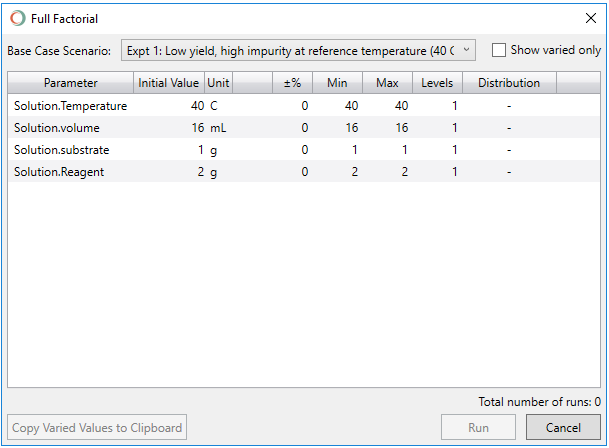Select your base case (or centre-point) scenario using the dropdown menu, where it says Base Case Scenario. Below this, you will see 9 columns:

• Parameter: This contains the names of your scenario factors
• Initial Value: This contains the base case value for the factor (from the scenarios sheet)
• Unit: This is the unit of the factor given in the scenarios sheet
• +/-%: This is an optional percentage amount by which you would like to vary the factor
• Min: This is an optional minimum value for a range of values for that factor
• Max: This is an optional maximum value for a range of values for that factor
• Levels: This is the number of levels over which you would like to vary each factor
• Distribution: This is type of spacing of levels; options are linear or logarithmic spacing.

Once you have selected the factors to vary, you can check the box beside Show varied only in the top right hand corner to show only the varied factors: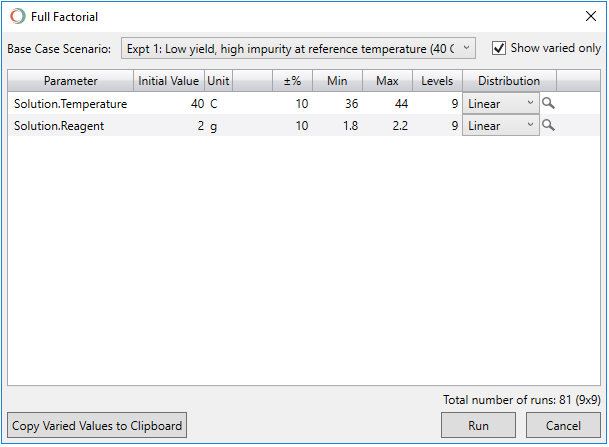At the bottom of this screen, press Run to run the simulations. This will run the Full Factorial exploration where your variations of the Base Case Scenario will be simulated.

You can also Copy the Varied Values to the Clipboard or Cancel.

Running the Full Factorial

Dynochem automation will run your simulations and this may take several seconds to minutes, depending on your model and how many scenarios you run. Once completed, a new tab called Full Factorial will be created in your Excel workbook.  In the above example, there are 81 runs composed of one base case and 80 variations of this. See below for a screenshot of the Base Case with the first 19 variations: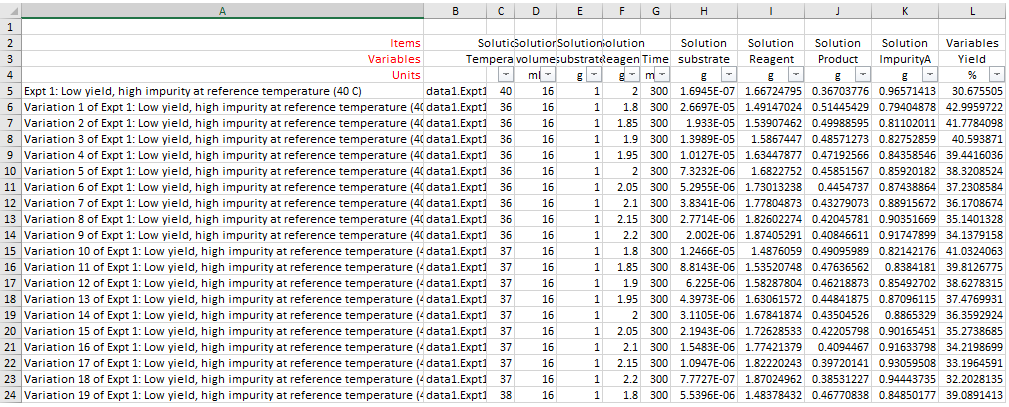On this worksheet:

• Column A: contains the names of the the Base case variations. These are called Variation 1, Variation 2,....Variation n.
• Column B: is the reference to the datasheet for the scenario. This will be the same reference as the Base case.
• Column C-F: contain the input values / factor settings for each scenario.
• Column G-L: contain the end times of each scenario and the final values of all plotted responses.

It is common to visualize such results using a Response Surface.

Varying more than 2 factors using Full Factorial

In the example below (from the fit_gas model, an example of a hydrogenation reaction), Solution temperature, Solution cat and Gas H2 were all varied. The number of levels selected for each factor was 5. Therefore, the total number of runs is equal to 125.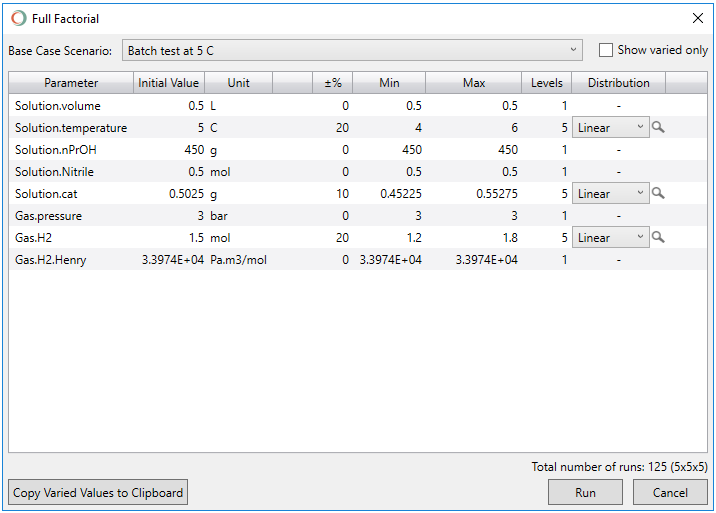You can select Show varied only. This will show the factors that are being varied only.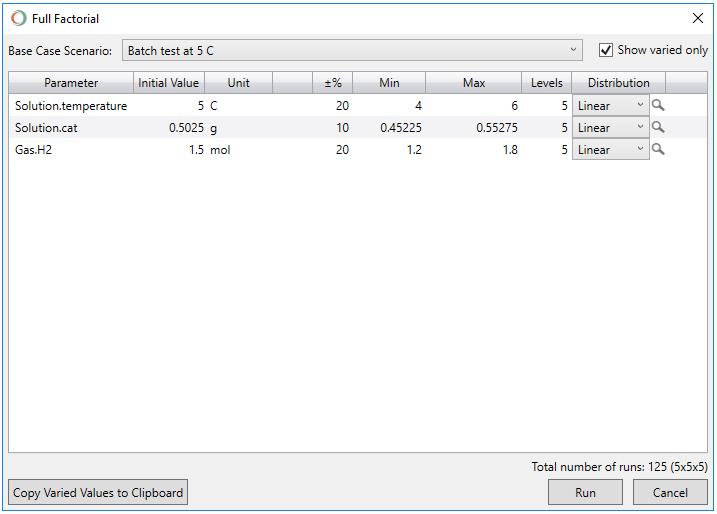Clicking on the Run button will start the automated simulation runs . Once completed, a new tab called Full Factorial will be created and placed in your Excel workbook.